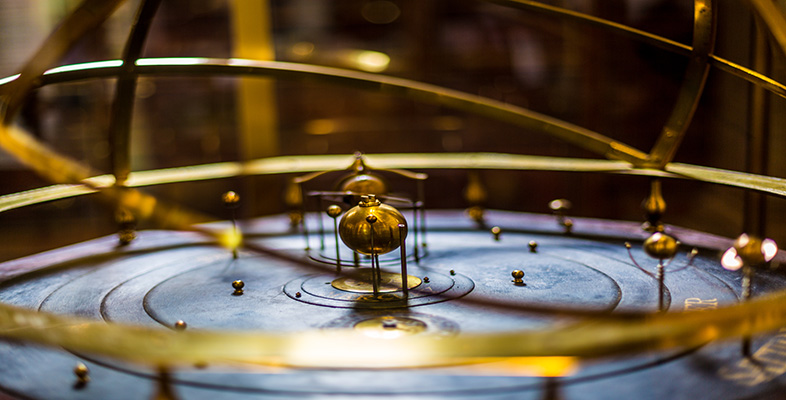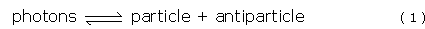Science, Maths & Technology

### Become an OU studentThe evolving Universe

Start this free course now. Just create an account and sign in. Enrol and complete the course for a free statement of participation or digital badge if available.

# 1.3 The very early Universe

Time: <10−36 s

Temperature: >1028 K

Energy: >3 × 1015 GeV (i.e. >3 × 1024 eV)

At the very earliest times in the history of the Universe, we can only presume that a superunification of the four interactions was in operation. Unfortunately, no reliable theory of superunification is yet available, so nothing can be said about the contents or behaviour of the Universe in its earliest moments. Indeed, it may even be that the concept of 'time' itself had no meaning until the Universe had cooled below a certain threshold.

The first stop on the tour where anything can be said is at about 3 × 10−44 s after the Big Bang - an epoch known as the Planck time. By this time the mean energy per particle in the Universe had fallen to around 1019 GeV. This is the energy at which the gravitational force on an individual particle has roughly the same strength as its other interactions. An idea of the typical size scale of the Universe can be gained by thinking about how far a photon of light could have travelled during this period. By the time the Universe was 3 × 10−44 s old, a beam of light travelling at 3 × 108 m s−1 could have travelled a distance of only about 10−35 m. This tiny dimension is referred to as the Planck length. This is the sort of scale on which the hidden dimensions involved in the theory of everything, known as M-theory, are supposed to be curled up very small.

## Question 2

How does the Planck length compare with the typical size of an atomic nucleus?

The typical size for an atomic nucleus is about 10−14 m. So the Planck length is around 1021 times smaller than an atomic nucleus. (It is as many times smaller than a nucleus as a nucleus is smaller than the Earth!)

As Figure 1 shows, at or around the Planck time, it is supposed that gravitational interactions became distinct from a grand unified interaction that included the three effects seen today as the electromagnetic, strong and weak interactions. In order to describe the gravitational interactions at these times a theory of quantum gravity is required. However no such theory is yet available.

The temperature, and hence the mean energy per particle, was far higher at this time than can be recreated in particle accelerators here on Earth. Cosmologists and particle physicists can therefore only speculate on what might have occurred in the very early Universe. The best guess is that pairs of matter and antimatter particles of all types were spontaneously created out of pure energy, which can be thought of as a 'sea' of photons filling the entire Universe. With equal spontaneity, pairs of matter and antimatter particles also combined with each other again to produce photons. The overall processes of pair creation (left to right) and annihilation (right to left) can be represented as:At the temperatures existing in the Universe today, reactions such as this proceed preferentially from right to left. However, at the temperatures applying in the early Universe, the reactions proceeded in both directions at the same rate, for all types of particle. A stable situation was reached in which the rates of pair creation and annihilation exactly balanced, and equal amounts of matter/antimatter and radiation were maintained.

As well as quarks and leptons, if the Grand Unified Theory is correct, then this is when the particles known as X bosons would also have been in evidence. These particles are the quanta of the grand unified interaction and are suggested as a means of converting between quarks and leptons, or between matter and antimatter.

The next stop in time is at about 10−36 s after the Big Bang when the Universe had a temperature of about 1028 K. This temperature marks the energy at which the strong interactions became distinct from the electroweak interactions (see Figure 1).

## Question 3

How long after the Planck time did the strong and electroweak interactions become distinct?